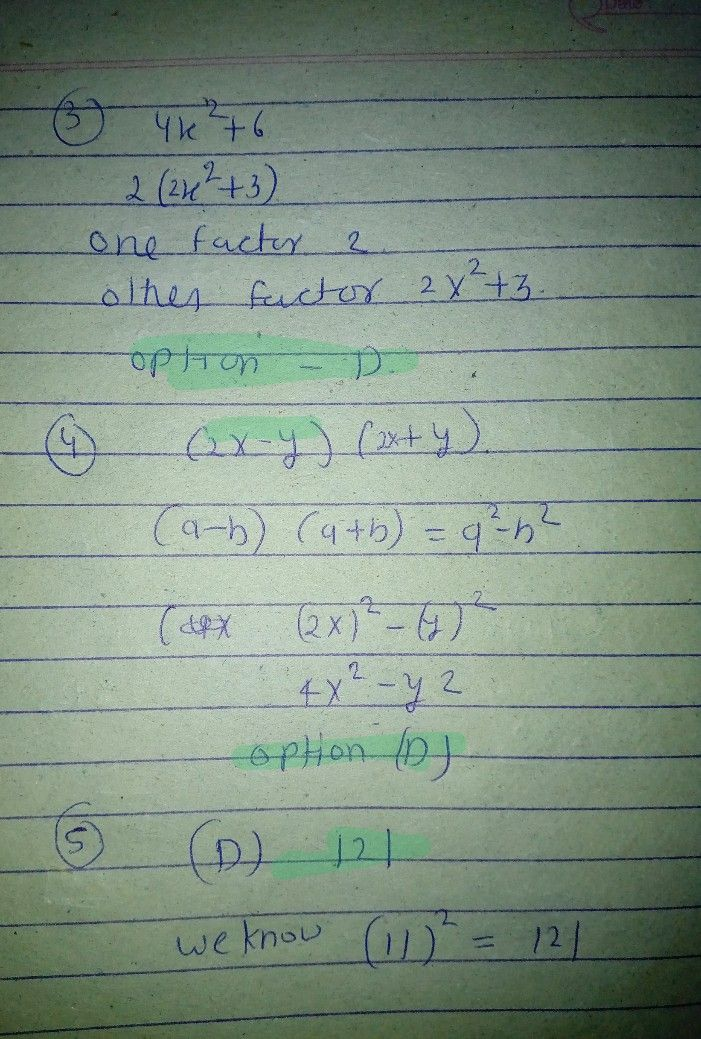Symbol
ProblemATULTIPLE CHOICE $2$ $mcc1=0$ Choose the letter of the correct answer. Write your answer on the space providet I What is the process of finding the factors of an expression which is the reverse process of multiplication? A. factoring B. rationalization C. special product D. continuous division 2. Which of the following pair of numbers has a $Ocro162$ $p$ $12an$ 18 A. 2 and 3 B. 8 and 12 C. 6 and 10 3. If one factor oBf . $4x^{2.6ts2}$ $4x.6$ what is t2hx e o6 ther factor? A. 2x 3 C. D. 2x 3 4. Which of the following expressions has $lac1ors$ $y^{2}$ (2x- $y$ $an1\left(2x$ $0$ $4x2-y$ y? A. 2x+ ya B. 2x- ya C. 4x $ng1s$ perfecCt s. q12u5 are number? 5. Which of the $o1omin$ $24$ $D.121$ A. 8 B. 6. All of the following expressions are perfect squares, $xCE\pi$ $D.25xy$ one. What is it? A. 12a-b B. xy C. 16a b 7. Which of the following is equal $tox^{2-6x+9y^{27}}$ $c.\left(2x-3y\right)^{2}$ $D.\left(2x43y\right)$ A. [x-3yP B. $x+3y\right)$ 8. Which of the following terms is $1cscnbx$ as "the ratio of two polynomial expressions ? lincar algebraic expression C. rational algebraic expression AB. . lincar algebraic equation D. rational algebraic equation 9. In a rational algebraic expression written in the where $Pand0ar$ $fomof^{E}$ $9$ $a1t0\bar{D.2}$ polynomials, the polynomial Q must not be equal A.-1 B. 0 C. 1 10. Which of the following is considered a rational algebraic expression? 4x+11 A 3n B. 3n-n+ 2 9 C. $2x^{-2}-7$ $-$ D. n--1 11. Which of the $olo$ $ing1s$ an example of a rational algebraic expression? 3n A. 15 V $B.\dfrac {3n+\sqrt{n} } {2+3n}$ C. Sx'+4 D. 2n-241 2n+3 12. Evaluate the expression 32rx +5 when x- 1. A. -1 B. 0 C. 3 D. 4 13. Which of the following is one of the steps in simplifying rational expressions? A. Add the common factors. B. Subtract out the common factors. DC. . Multiply the common factors. Divide out the common factors.
7th-9th grade
Other
Search count: 169
SolutionQanda teacher - RASHMIKAthanks for asking a good question
We are only one question allow at time by qanda policy
if you have more questions so pls ask on preferred match on my profile
thanksStudent
thankyouQanda teacher - RASHMIKA
welcome
please evaluate ansStudent
but it is multiple choice
and how about the other number?Qanda teacher - RASHMIKA
what